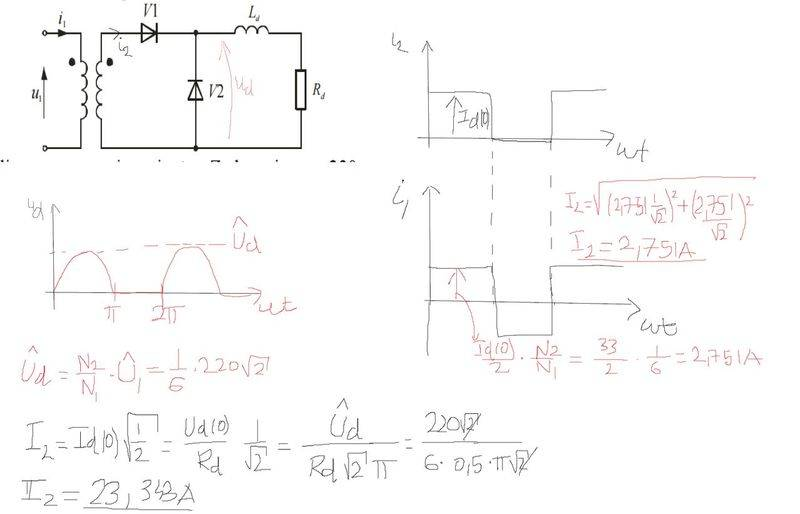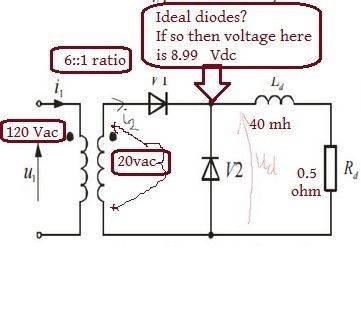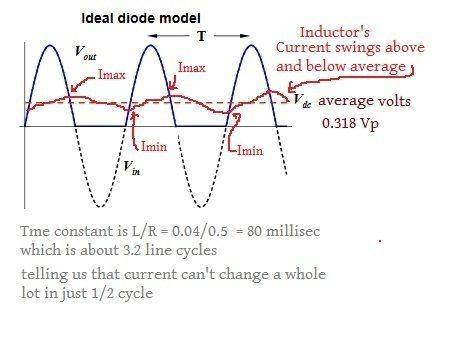# Half wave rectifier with ideal transformer

Ivan Antunovic

## Homework Statement

Determine the rms values of the currents of primary and secondary windings of transformer in the network
circuit diagram. Transformer is perfect, with ratio 6: 1,and negligible
AC component of magnetizing current, is connected to the sinus supply network U1 = 220 V,
f = 50 Hz.Ld = 40 mH, R = 0.5 Ω

## The Attempt at a Solution[/B]
My questions is how did we get square wave forms for the currents i1 and i2 , it really confuses me( I found waveforms in the book) .Shouldn't current i1 be a sine current with 90 angle delay behind voltage source.

## Answers and Replies

Homework Helper
Gold Member
My questions is how did we get square wave forms for the currents i1 and i2

Is because the inductance is very large compared to the resistance. If you calculate the time constant L/R, it comes out to be 80ms, which means the sinusoidal steady state will be reached in 400ms (5*time constant), which takes 20 cycles of input voltage. Here, at the beginning of every cycle, a new transient starts, hence the inductor never really attains a steady state. The transient exponential behavior of the current can be approximated to a square wave since rate of change of current is small due to high time constant. Also, current through Rd can be approximated to constant dc.

Last edited:
Ivan Antunovic
Is because the inductance is very large compared to the resistance. If you calculate the time constant L/R, it comes out to be 80ms, which means the sinusoidal steady state will be reached in 400ms (5*time constant), which takes 20 cycles of input voltage. Here, at the beginning of every cycle, a new transient starts, hence the inductor never really attains a steady state. The transient exponential behavior of the current can be approximated to a square wave since rate of change of current is small due to high time constant. Also, current through Rd can be approximated to constant dc.
But the how do you explain current i1?

Homework Helper
Gold Member
But the how do you explain current i1?
I1 is reflection of i2. So, when i2 becomes 0, i1 should also be zero.

Last edited:
•Ivan Antunovic
Ivan Antunovic
I1 is reflection of i2. So, when i2 becomes 0, i1 should also be zero.
Thank you for your answer , transformers are really my weak spot :/

Homework Helper
Gold Member
This circuit is very hard to analyze not because of the transformer but because the diodes introduce nonlinear behavior. This problem is best (probaly solely) solved with software like SPICE.

Ivan Antunovic
This circuit is very hard to analyze not because of the transformer but because the diodes introduce nonlinear behavior. This problem is best (probaly solely) solved with software like SPICE.
Yes nonilinearity of the diodes produces really non intuitive waveforms

Gold Member
Dearly Missed
The academic way is to write differential equations and solve for i(t) , which defines both the startup transient and steady state.

Steady state is a lot easier

i think in pictures and figure out the formulas
here's an approach to get you started

examine the circuit
can we assume ideal diodes too ?
What do we know? I wrote it in brown.Ideal diodes allow no forward voltage drop so we get at junction of the diodes perfect half wave rectified 20Vac and i assumed RMS.
But since it's now DC (lumpy but unidirectional) we should switch to average
average of a halfwave rectified sinewave is 0.318Vpeak, see http://my.ece.ucsb.edu/York/Bobsclass/2B/Extras/Half-Wave Rectifier.pdf
and Vpeak is VRMS X √2

20 X √2 V 0.318 = 8.99 (to slide rule accuracy of 3 figures)

and that'll be the average voltage at junction of diodes and Ld.

Now an inductor cannot oppose DC beyond a few time constants so the average voltage at other side of Ld must also be 8.99 volts
meaning average current through Ld and Rd must be by Ohm's law 8.99 /0.5 = 18.0 amps
During positive half cycles the current increases to a value above average
during negative half cycles it decreases to a value below average
with a time constant L/r = ##\frac {0.04} {0.5} ## = 0.08 secBetween positive half cycles , while current is decreasing, , i = imax et/0.08
in 30 miliseconds , just a little longer than 1/2 cycle, it'll decrease to Imin = ~68% of whatever Imax was, 32% decrease
so we can estimate that current swings between Imax and Imin , roughly Iavg ±16% , as sketched above.

Of course Ohm's Law dictates voltage across Rd must do exactly the same.

.......................................................
Why the circuit works:
During positive half cycles the inductor absorbs energy from the supply and transformer
during negative half cycles the inductor delivers some of its absorbed energy to Rd

Of course at startup, current will start from zero and increase during every positive half cycle, after several cycles approaching this steady state sawtooth looking waveform , ±16% about average .

.....................................................

Now you have an intuitive :"Sanity Check" method to compare against whatever Pspice (or your differential equation) tells you.
That's how i always had to flush out my algebra mistakes, and later on when QBasic and computers replaced my slide rule, my programming errors .
..............................................................................................................................

Your homework circuit is the basis of many kinds of regulators,
both simple ones like we just analyzed and
complex ones where they use switching transistors instead of diodes, so called "Switch Mode Power Supplies" ...

So it's important that you get accustomed to working it in your head .

Doubtless your math is fifty years more fresh than mine so i wouldn't presume to lecture you there.
I offer this "simple thinking" approach to complement your math skill. Maybe it'll help you spot an impossible result before it propagates through an entire derivation.

Hope it helps.

old jim

Last edited: